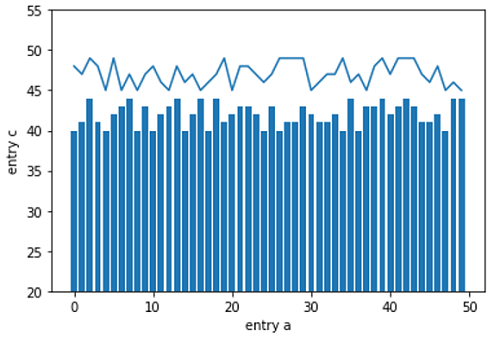# Python | Plotting using Dictionary

In this tutorial, we are going to learn how to plot data which is stored in a dictionary?
Submitted by Anuj Singh, on August 06, 2020

Sometimes, data is available in the format of a Dictionary and we can use it directly to plot in matplotlib without converting it into separate lists.

The following example is an illustration of how to plot using Dictionary.

Illustration:## Python code for plotting using dictionary

```import numpy as np
import matplotlib.pyplot as plt

data = {'a': np.arange(50),
'c': np.random.randint(45, 50, 50),
'd': np.random.randint(40,45,50)}

plt.plot('a', 'c', data=data)
plt.bar('a', 'd', data=data)
plt.ylim(20,55)
plt.xlabel('entry a')
plt.ylabel('entry c')
plt.show()
```

Output:

```Output is as figure
```